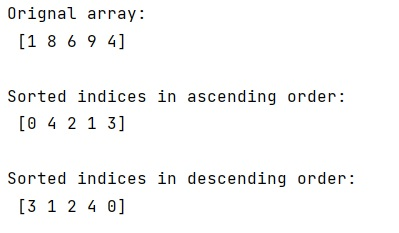# Is it possible to use numpy.argsort() in descending order?

Learn, whether is it possible to use numpy.argsort() in descending order? By Pranit Sharma Last updated : May 25, 2023

NumPy is an abbreviated form of Numerical Python. It is used for different types of scientific operations in python. Numpy is a vast library in python which is used for almost every kind of scientific or mathematical operation. It is itself an array which is a collection of various methods and functions for processing the arrays.

## numpy.argsort() Method

The numpy.argsort() method is used to get the sorted indices of a NumPy array, we need to understand that is it possible to use argsort() method in descending order.

If we negate an array, the lowest elements become the highest elements and the highest elements become the lowest elements.

Therefore, the indices of the N highest elements can be accessed by negating the result of argsort() method.

Another way to reason about this is to observe that the big elements are coming last in the argsort(). So, we can read from the end elements of the argsort() to find the N highest elements by using [::-1] with this method.

Let us understand with the help of an example,

## Python code to use numpy.argsort() in descending order

```# Import numpy
import numpy as np

# Creating an empty numpy array
arr = np.array([1, 8, 6, 9, 4])

# Display original array
print("Orignal array:\n",arr,"\n")

# 5 Sorted indices in ascending order
res_asc = arr.argsort()[:5]

# Display sorted indices in ascending order
print("Sorted indices in ascending order:\n",res_asc,"\n")

# 5 sorted indices i descending order
res_asc = (-arr).argsort()[:5]

# Display sorted indices in descending order
print("Sorted indices in descending order:\n",res_asc)
```

Output: